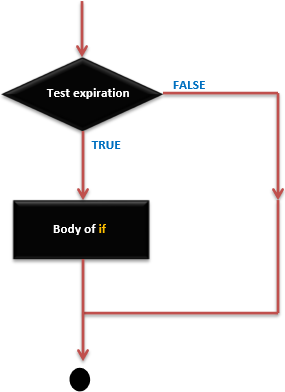# Arduino - If statement

It takes an expression in parenthesis and a statement or block of statements. If the expression is true then the statement or block of statements gets executed otherwise these statements are skipped.

## Different forms of if statement

Form 1

```if (expression)
statement;
```

You can use the if statement without braces { } if you have one statement.

Form 2

```if (expression) {
Block of statements;
}
```

## if Statement – Execution Sequence### Example

```/* Global variable definition */
int A = 5 ;
int B = 9 ;

Void setup () {

}

Void loop () {
/* check the boolean condition */
if (A > B) /* if condition is true then execute the following statement*/
A++;
/* check the boolean condition */
If ( ( A < B ) && ( B != 0 )) /* if condition is true then execute the following statement*/ {
A += B;
B--;
}
}
```
arduino_control_statements.htm